# Polynomials

Go back to  'Algebra Basics'

## Introduction to Polynomials

We at Cuemath believe that the easiest, most organic way to understand a concept is to be able to visualize it. So, this is what a polynomial looks like.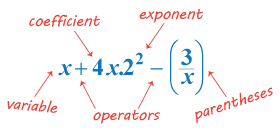## The Big Idea: What is a polynomial?

As we saw above, a polynomial is the parent term used to describe a certain type of algebraic expressions that contain variables, constants, and involve the operations of addition, subtraction, multiplication and division along with only positive powers associated with the variables.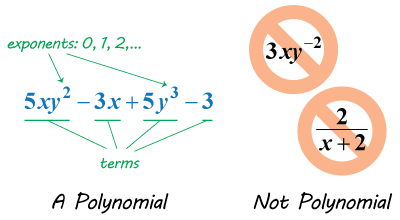### Degree of a polynomial

Think back to the components of a polynomial, as the name suggests, the degree of a polynomial is determined by the highest power a variable is raised to. Take a look at the following example: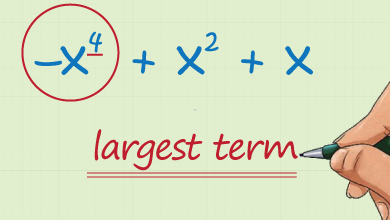## Why are polynomials important?

Polynomials form a large group of algebraic expressions. Any expression with only positive powers of the variables are termed as polynomials. As they cover such a huge chunk of all algebraic expressions, they tend to have a wide variety of applications.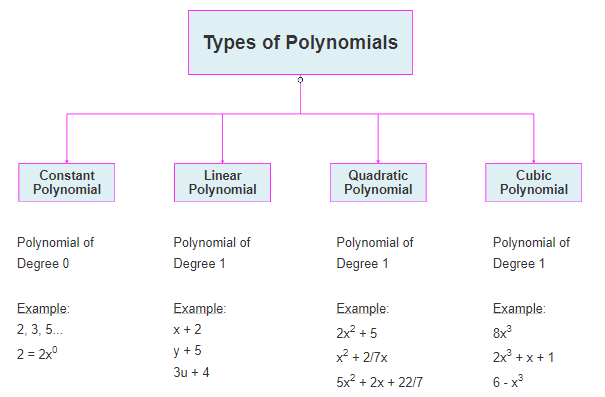One of those being very important for coordinate geometry. A lot of polynomials describe curves and understanding their properties is very important for a lot of higher mathematics. Here, have a look for yourself: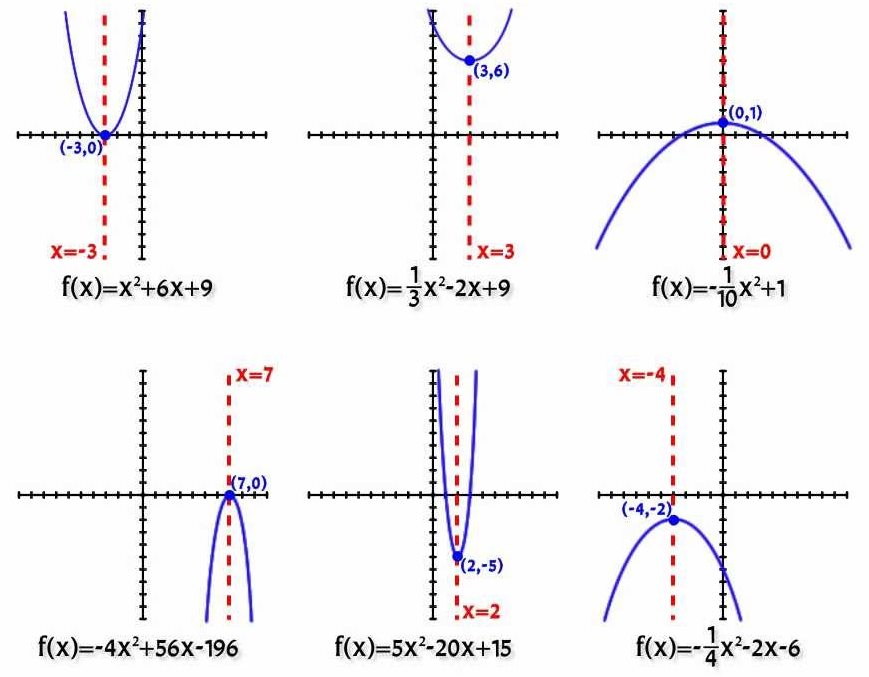### Addition and subtraction of polynomials

Whether you wish to add numbers together or you wish to add polynomials, the basic rules remain the same. The only difference is that when you are adding 34 to 127, you align the appropriate place values and carry the operation out. However, when dealing with the addition and subtraction of polynomials, one needs to pair up like terms and then add them up. Otherwise all the rules of addition and subtraction from numbers translate over to polynomials.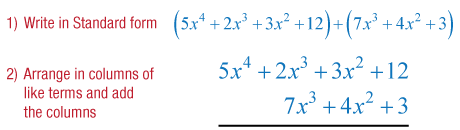### Multiplication and division of polynomials

For multiplication of polynomials, there are three laws which are to be kept in mind – distributive law, associative law and commutative law. For division, the most common method used to divide one polynomial by another is the long division method.